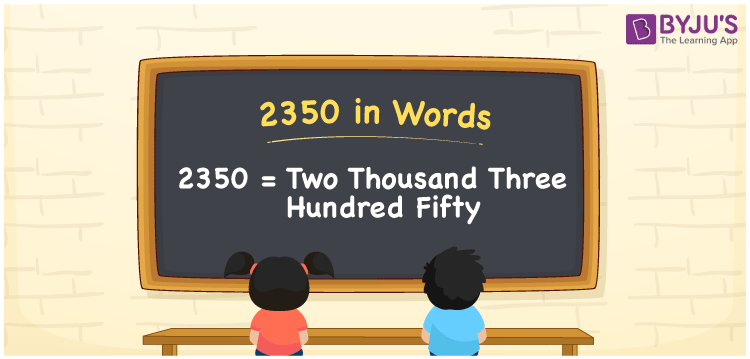# 2350 in words

2350 in words is written as Two Thousand Three Hundred and Fifty. 2350 represents the count or value. The article on Counting Numbers can give you an idea about count or counting. The number 2350 is used in expressions that relate to money, distance, length, year and others. Let us consider an example for 2350. “I wonder how advanced technology will be in the year Two Thousand Three Hundred and Fifty”. Another example, “A special dress was designed by a brand which had Two Thousand Three Hundred and Fifty golden beads embedded in it.”

 2350 in words Two Thousand Three Hundred and Fifty Two Thousand Three Hundred and Fifty in Numbers 2350

## 2350 in English Words## How to Write 2350 in Words?

We can convert 2350 to words using a place value chart. The number 2350 has 4 digits, so let’s make a chart that shows the place value up to 4 digits.

 Thousands Hundreds Tens Ones 2 3 5 0

Thus, we can write the expanded form as:

2 × Thousand + 3 × Hundred + 5 × Ten + 0 × One

= 2 × 1000 + 3 × 100 + 5 × 10 + 0 × 1

= 2350

= Two Thousand Three Hundred and Fifty.

2350 is the natural number that is succeeded by 2349 and preceded by 2351.

2350 in words – Two Thousand Three Hundred and Fifty.

Is 2350 an odd number? – No.

Is 2350 an even number? – Yes.

Is 2350 a perfect square number? – No.

Is 2350 a perfect cube number? – No.

Is 2350 a prime number? – No.

Is 2350 a composite number? – Yes.

## Solved Example

1. Write the number 2350 in expanded form

Solution: 2 × 1000 + 3 × 100 + 5 × 10 + 0 × 1.

We can write 2350 = 2000 + 300 + 50 + 0

= 2 × 1000 + 3 × 100 + 5 × 10 + 0 × 1.

## Frequently Asked Questions on 2350 in words

Q1

### How to write 2350 in words?

2350 in words is written as Two Thousand Three Hundred and Fifty.
Q2

### State whether True or False. 2350 is divisible by 3?

False. 2350 is not divisible by 3.
Q3

### Is 2350 divisible by 10?

Yes. 2350 is divisible by 10.